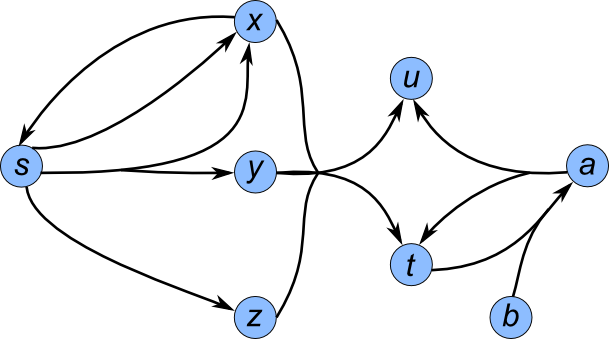View on GitHub

# halp

## Hypergraph Algorithms Package

Download this project as a .zip file Download this project as a tar.gz file

halp is a Python software package that provides both a directed and an undirected hypergraph implementation, as well as several important and canonical algorithms that operate on these hypergraphs.

Use pip to install the latest stable release with: `pip install halp`

Latest Stable Release: 1.0 - October 8, 2014

# Getting Started

What are directed and undirected hypergraphs?

Simply put, hypergraphs are a generalization of graphs.

A directed hypergraph contains nodes and hyperedges. Each hyperedge connects a tail set of nodes to a head set of nodes (where the tail and head cannot both be empty). A directed edge in a traditional directed graph, where an edge connects from exactly one node to exactly one other node, is a special case of a directed hyperedge.

Similarly, an undirected hypergraph contains nodes and hyperedges. The difference here is that each hyperedge connects a single set of nodes together. An undirected edge in a traditional undirected graph, where two edges are connected to each other, is a special case of an undirected hyperedge.

### Using Directed Hypergraphs

The following examples for directed hypergraphs will use the following hypergraph:We can create a hypergraph explicitly:

```from halp.directed_hypergraph import DirectedHypergraph

# Initialize an empty hypergraph
H = DirectedHypergraph()

# Add nodes 's' and 't' individually with arbitrary attributes
H.add_node('s', source=True)
H.add_node('t', sink=True)
# Add several nodes simultaneously, having the same arbitrary attributes
H.add_nodes(['x', 'y', 'z', 'u', 'a', 'b'], label='grey')

# Add hyperedge from {'s'} to {'x'} with a weight of 1
H.add_hyperedge(set(['s']), set(['x']), weight=1)
# Add hyperedge from {'s'} to {'x', 'y'} with some arbitrary attributes and weight of 2
H.add_hyperedge(set(['s']), set(['x', 'y']), {'color': 'red', 'active': True}, weight=2)
# Add several hyperedges simultaneously, having individual weights
hyperedges = [(['s'], ['z'], {'weight': 2}),
(['s'], ['t'], {'weight': 100}),
(['x'], ['s'], {'weight': 1}),
(['x', 'y', 'z'], ['u', 't'], {'weight': 3}),
(('t', 'b'), ('a'), {'weight': 1}),
(set(['a']), set(['u', 't']), {'weight': 1})]
H.add_hyperedges(hyperedges)

# Note: a hyperedge can be added even if it contains nodes that haven't
# previously been put into the graph; the library will automatically add them!
```

If a hypergraph is stored in a file similar to:

``````tail;head;weight
s;x;1
s;y,x;2
s;z;2
s;t;100
x;s;1
z,y,x;u,t;3
a;u,t;1
b,t;a;1
``````

then we can read the hypergraph from the file with:

```from halp.directed_hypergraph import DirectedHypergraph

# Initialize an empty hypergraph
H = DirectedHypergraph()

node_delimiter = ','
column_separator = ';'

H.read("hypergraph_filename.txt", node_delimiter, column_separator)
```

Finally, we can execute an algorithm on an initialized hypergraph:

```from halp.directed_hypergraph import DirectedHypergraph
from halp.algorithms import directed_paths
H = DirectedHypergraph()
H.read("hypergraph_filename.txt", ',', ';')

# Execute the "Shortest Sum B-Tree" algorithm with root 's'
Pv, W, ordering = \
directed_paths.shortest_b_tree(H,
's',
directed_paths.sum_function,
True)
# Use the returned result to retrieve the hypertree (a subhypergraph)
sub_H = directed_paths.get_hypertree_from_predecessors(H, Pv, 's', W)
```

### Testing

Unit tests for the package are located in `tests/`, and can be run with `python setup.py test`.

The test runner depends on pytest.

Travis CI checks for pep8 compliance on all code. If the code doesn't meet pep8 compliance, the test suite will report a failure.